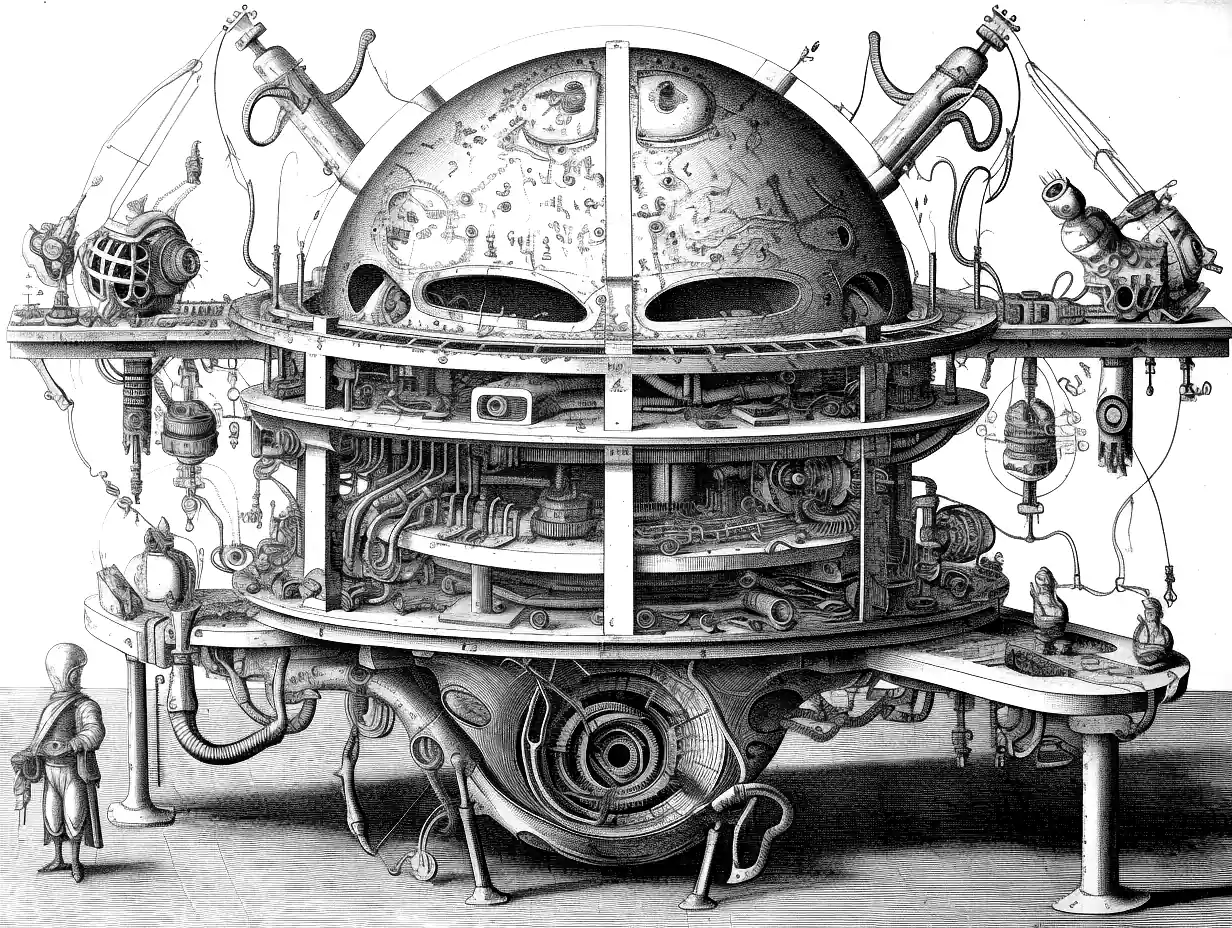# Matrix square roots

## Whitening, preconditioning etc

### Assumed audience:Interpretations and tricks for matrix square roots. TBC

## Low-rank-plus-diagonal roots

Perturbations of low rank matrices have non-low-rank roots, but there exist efficient algorithms for findimg them at least. Fasi, Higham, and Liu (2023):

We consider the problem of computing the square root of a perturbation of the scaled identity matrix, $$\mathrm{A}=\alpha \mathrm{I}_n+\mathrm{U} \mathrm{V}^{H}$$, where $$\mathrm{U}$$ and $$\mathrm{V}$$ are $$n \times k$$ matrices with $$k \leq n$$. This problem arises in various applications, including computer vision and optimization methods for machine learning. We derive a new formula for the $$p$$ th root of $$\mathrm{A}$$ that involves a weighted sum of powers of the $$p$$ th root of the $$k \times k$$ matrix $$\alpha \mathrm{I}_k+\mathrm{V}^{H} \mathrm{U}$$. This formula is particularly attractive for the square root, since the sum has just one term when $$p=2$$. We also derive a new class of Newton iterations for computing the square root that exploit the low-rank structure.

Their method works for low-rank-plus-diagonal matrices without negative eigenvalues.

Theorem: Let $$\mathrm{U}, \mathrm{V} \in \mathbb{C}^{n \times k}$$ with $$k \leq n$$ and assume that $$\mathrm{V}^{H} \mathrm{U}$$ is nonsingular. Let $$f$$ be defined on the spectrum of $$\mathrm{A}=\alpha \mathrm{I}_n+\mathrm{U} \mathrm{V}^{H}$$, and if $$k=n$$ let $$f$$ be defined at $$\alpha$$. Then $\quad f(\mathrm{A})=f(\alpha) \mathrm{I}_n+\mathrm{U}\left(\mathrm{V}^{H} \mathrm{U}\right)^{-1}\left(f\left(\alpha \mathrm{I}_k+\mathrm{V}^{H} \mathrm{U}\right)-f(\alpha) \mathrm{I}_k\right) \mathrm{V}^{H}.$ The theorem says two things: that $$f(\mathrm{A})$$, like $$\mathrm{A}$$, is a perturbation of rank at most $$k$$ of the identity matrix and that $$f(\mathrm{A})$$ can be computed by evaluating $$f$$ and the inverse at two $$k \times k$$ matrices.

I would call this a generalized Woodbury formula, and I think it is pretty cool; which tells you something about my current obsession profile. Anyway, they use it to discover the following:

Let $$\mathrm{U}, \mathrm{V} \in \mathbb{C}^{n \times k}$$ with $$k \leq n$$ have full rank and let the matrix $$\mathrm{A}=\alpha \mathrm{I}_n+\mathrm{U} \mathrm{V}^{H}$$ have no eigenvalues on $$\mathbb{R}^{-}$$. Then for any integer $$p \geq 1$$, $\mathrm{A}^{1 / p}=\alpha^{1 / p} \mathrm{I}_n+\mathrm{U}\left(\sum_{i=0}^{p-1} \alpha^{i / p} \cdot\left(\alpha \mathrm{I}_k+\mathrm{V}^{H} \mathrm{U}\right)^{(p-i-1) / p}\right)^{-1} \mathrm{V}^{H}$

and in particular

Let $$\mathrm{U}, \mathrm{V} \in \mathbb{C}^{n \times k}$$ with $$k \leq n$$ have full rank and let the matrix $$\mathrm{A}=\alpha \mathrm{I}_n+\mathrm{U} \mathrm{V}^{H}$$ have no eigenvalues on $$\mathbb{R}^{-}$$. Then $\mathrm{A}^{1 / 2}=\alpha^{1 / 2} \mathrm{I}_n+\mathrm{U}\left(\left(\alpha \mathrm{I}_k+\mathrm{V}^{H} \mathrm{U}\right)^{1 / 2}+\alpha^{1 / 2} \mathrm{I}_k\right)^{-1} \mathrm{V}^{H}.$

They also derive an explicit gradient step for calculating it, namely Denman-Beaver iteration:

The (scaled) DB iteration is \begin{aligned} \mathrm{X}_{i+1} & =\frac{1}{2}\left(\mu_i \mathrm{X}_i+\mu_i^{-1} \mathrm{Y}_i^{-1}\right), & \mathrm{X}_0=\mathrm{A}, \\ \mathrm{Y}_{i+1} & =\frac{1}{2}\left(\mu_i \mathrm{Y}_i+\mu_i^{-1} \mathrm{X}_i^{-1}\right), & \mathrm{Y}_0=\mathrm{I}, \end{aligned} where the positive scaling parameter $$\mu_i \in \mathbb{R}$$ can be used to accelerate the convergence of the method in its initial steps. The choice $$\mu_i=1$$ yields the unscaled DB method, for which $$\mathrm{X}_i$$ and $$\mathrm{Y}_i$$ converge quadratically to $$\mathrm{A}^{1 / 2}$$ and $$\mathrm{A}^{-1 / 2}$$, respectively.

… for $$i \geq 0$$ the iterates $$\mathrm{X}_i$$ and $$\mathrm{Y}_i$$ can be written in the form \begin{aligned} & \mathrm{X}_i=\beta_i \mathrm{I}_n+\mathrm{U} \mathrm{B}_i \mathrm{V}^{H}, \quad \beta_i \in \mathbb{C}, \quad \mathrm{B}_i \in \mathbb{C}^{k \times k}, \\ & \mathrm{Y}_i=\gamma_i \mathrm{I}_n+\mathrm{U} \mathrm{C}_i \mathrm{V}^{H}, \quad \gamma_i \in \mathbb{C}, \quad \mathrm{C}_i \in \mathbb{C}^{k \times k} . \\ & \end{aligned}

This gets us a computational speedup, although of a rather complicated kind. For a start its constant factor is very favourable compared to the naive approach, but it also has a somewhat favourable scaling with $$n$$, being less-than-cubic although more than quadratic depending on some optimisation convergence rates, which depends both on the problem and upon optimal selection of $$\beta_i,\gamma_i$$, which they give a recipe for but it gets kinda complicated and engineering-y.

Anyway, let us suppose $$\mathrm{U}=\mathrm{V}=\mathrm{Z}$$ and replay that. Then

$\mathrm{A}^{1 / 2}=\alpha^{1 / 2} \mathrm{I}_n+\mathrm{Z}\left(\left(\alpha \mathrm{I}_k+\mathrm{Z}^{H} \mathrm{Z}\right)^{1 / 2}+\alpha^{1 / 2} \mathrm{I}_k\right)^{-1} \mathrm{Z}^{H}.$

The Denman-Beaver step becomes

\begin{aligned} \mathrm{X}_{i+1} & =\frac{1}{2}\left(\mu_i \mathrm{X}_i+\mu_i^{-1} \mathrm{Y}_i^{-1}\right), & \mathrm{X}_0=\mathrm{A}, \\ \mathrm{Y}_{i+1} & =\frac{1}{2}\left(\mu_i \mathrm{Y}_i+\mu_i^{-1} \mathrm{X}_i^{-1}\right), & \mathrm{Y}_0=\mathrm{I}, \end{aligned}

This looked useful but now I note that this is giving us a full-size square root, rather than a low-rank square root, which is not helpful to me.

### No comments yet. Why not leave one?

GitHub-flavored Markdown & a sane subset of HTML is supported.